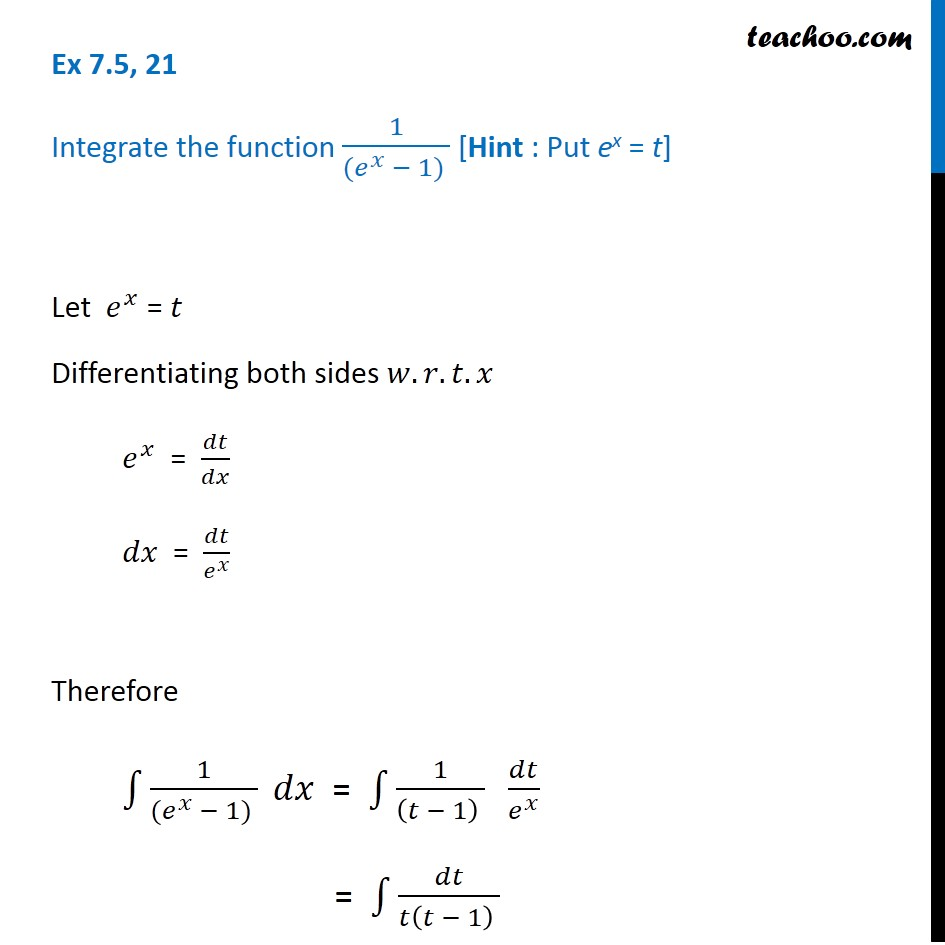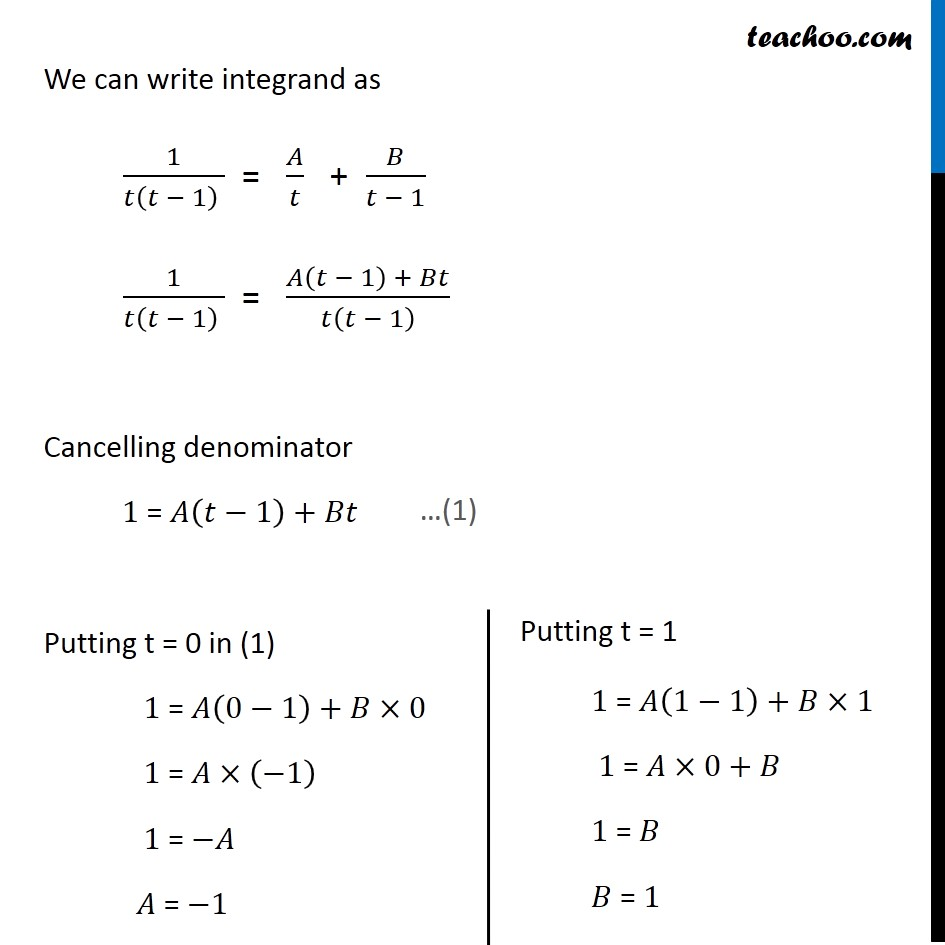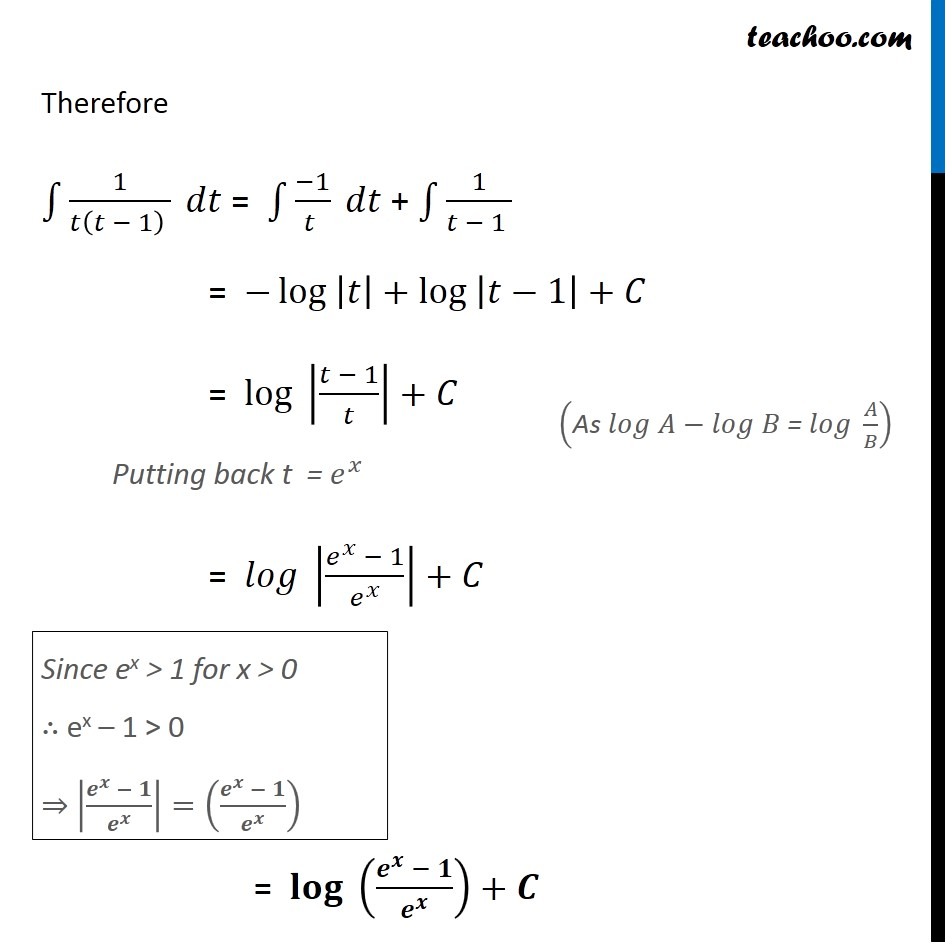Integration by partial fraction - Type 1

Chapter 7 Class 12 Integrals
Concept wiseIntroducing your new favourite teacher - Teachoo Black, at only ₹83 per month

### Transcript

Ex 7.5, 21 Integrate the function 1/((𝑒^𝑥 − 1) ) [Hint : Put ex = t] Let 𝑒^𝑥 = 𝑡 Differentiating both sides 𝑤.𝑟.𝑡.𝑥 𝑒^𝑥 = 𝑑𝑡/𝑑𝑥 𝑑𝑥 = 𝑑𝑡/𝑒^𝑥 Therefore ∫1▒1/((𝑒^𝑥 − 1) ) 𝑑𝑥 = ∫1▒1/((𝑡 − 1) ) 𝑑𝑡/𝑒^𝑥 = ∫1▒𝑑𝑡/(𝑡(𝑡 − 1) ) We can write integrand as 1/(𝑡(𝑡 − 1) ) = 𝐴/𝑡 + 𝐵/(𝑡 − 1) 1/(𝑡(𝑡 − 1) ) = (𝐴(𝑡 − 1) + 𝐵𝑡)/𝑡(𝑡 − 1) Cancelling denominator 1 = 𝐴(𝑡−1)+𝐵𝑡 Putting t = 0 in (1) 1 = 𝐴(0−1)+𝐵×0 1 = 𝐴×(−1) 1 = −𝐴 𝐴 = −1 Putting t = 1 1 = 𝐴(1−1)+𝐵×1 1 = 𝐴×0+𝐵 1 = 𝐵 𝐵 = 1 Therefore ∫1▒1/(𝑡(𝑡 − 1) ) 𝑑𝑡 = ∫1▒(−1)/(𝑡 ) 𝑑𝑡 + ∫1▒1/(𝑡 − 1 ) = −〖log 〗⁡|𝑡|+〖log 〗⁡|𝑡−1|+𝐶 = 〖log 〗⁡|(𝑡 − 1)/𝑡|+𝐶 Putting back t = 𝑒^𝑥 = 〖𝑙𝑜𝑔 〗⁡|(𝑒^𝑥 − 1)/𝑒^𝑥 |+𝐶 = 〖𝐥𝐨𝐠 〗⁡((𝒆^𝒙 − 𝟏)/𝒆^𝒙 )+𝑪 Since ex > 1 for x > 0 ∴ ex – 1 > 0 ⇒ |(𝒆^𝒙 − 𝟏)/𝒆^𝒙 |=((𝒆^𝒙 − 𝟏)/𝒆^𝒙 ) ("As " 𝑙𝑜𝑔 𝐴−𝑙𝑜𝑔 𝐵" = " 𝑙𝑜𝑔 𝐴/𝐵)